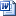# Math Worksheet Templates

Math is something fascinating for some people and for some people, it is something bothersome. But there are a lot of people who love math and try to make the younger generation love math too. Teachers are in charge of teaching children and in order to get the love for math grow, it is a good thing to start studying math from young age with the certain exercises of math those are fun for the children. The children must also being told about the easy ways to learn and study math by using the certain tools such as the abacus method of calculation or by using tables or worksheets.The worksheets are actually one of the easiest ways that will help children to develop knowledge of math. By using worksheets, children will need to fill out blank columns and rows those will need to be filled with the correct answer of the certain equations. The worksheets can also be used or exercise in math and to see just how far children understand about math so far. There are four worksheets of math calculation available, addition, division, multiplication and the subtraction worksheets. All of the worksheets are easy to be used and to be taught to children who are new with math

Math calculation worksheets are including the addition worksheet, division worksheet, multiplication worksheet and subtraction worksheet. Each one of the worksheet has eleven columns and eleven rows. One column and row has numbers from 1 to 10 and has a certain symbol of math calculation and the rest of rows and columns are empty.

This simple math worksheet templates are suitable for teachers or parents who just want to test their children on math. There are 10 x 10 blank cells that can be filled with correct answers by multiplying, adding, subtracting or dividing numbers from 1 to 10. Download and print it. Give your kids pens to calculating and filled all empty cells. Don’t forget to prepare gifts if they can fill them without mistakes.Math Worksheet - Division (35.5 KiB, 1,409 hits)Math Worksheet - Subtraction (35.5 KiB, 1,564 hits)Math Worksheet - Multiplication (35.5 KiB, 1,688 hits)Math Worksheet - Addition (35.5 KiB, 1,634 hits)

Math Worksheet Templates | | 4.5